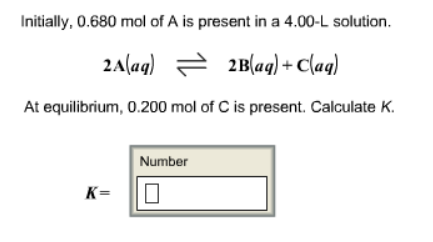# Problem: Initially, 0.680 mol of A is present in a 4.00-L solution. 2A (aq) ⇌ 2B (aq) + C (aq) At equilibrium, 0.200 mol of C is present. Calculate K.

###### FREE Expert Solution
93% (248 ratings)###### Problem Details

Initially, 0.680 mol of A is present in a 4.00-L solution.

2A (aq) ⇌ 2B (aq) + C (aq)

At equilibrium, 0.200 mol of C is present. Calculate K.